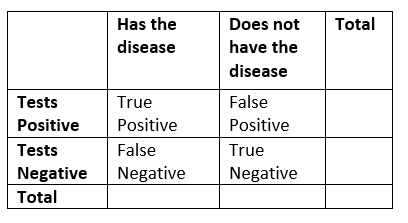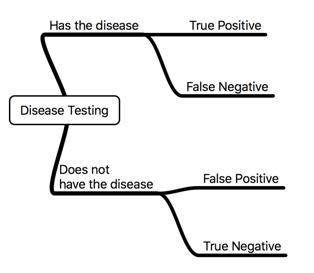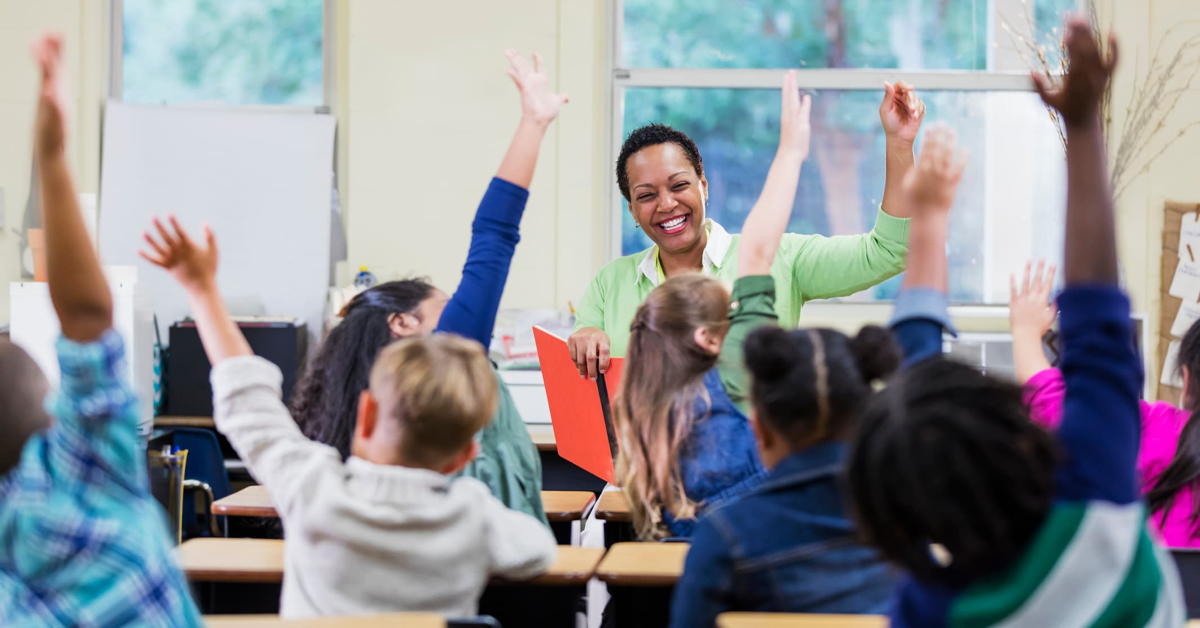# Probability

Lesson 8

Math

Unit 8

Lesson 8 of 10

## Objective

Make decisions about medical testing based on conditional probabilities.

## Common Core Standards

### Core Standards

• S.CP.A.3 — Understand the conditional probability of A given B as P(A and B)/P(B), and interpret independence of A and B as saying that the conditional probability of A given B is the same as the probability of A, and the conditional probability of B given A is the same as the probability of B.
• S.CP.A.4 — Construct and interpret two-way frequency tables of data when two categories are associated with each object being classified. Use the two-way table as a sample space to decide if events are independent and to approximate conditional probabilities. For example, collect data from a random sample of students in your school on their favorite subject among math, science, and English. Estimate the probability that a randomly selected student from your school will favor science given that the student is in tenth grade. Do the same for other subjects and compare the results.
• S.CP.A.5 — Recognize and explain the concepts of conditional probability and independence in everyday language and everyday situations. For example, compare the chance of having lung cancer if you are a smoker with the chance of being a smoker if you have lung cancer.

## Criteria for Success

1. Describe that no test for identifying a disease is perfect—there are times when a test records a positive result when there is no disease present and there are times when a test records a negative result when there is a disease present.
2. Use multiple representations (two-way frequency tables, tree diagrams, Venn diagrams, etc.) to visualize a probability situation with medical testing and use these visuals to determine probabilities.
3. Apply and develop probabilities from given sample sizes and associated data.
4. Identify which probabilities are conditional and which are not, thus determining independent and dependent variables.

## Tips for Teachers

In terms of pacing, this lesson should spread over two days due to the depth of this content.

Fishtank Plus

Unlock features to optimize your prep time, plan engaging lessons, and monitor student progress.

## Anchor Problems

In a certain population, 30% of people get a particular disease. A test was developed to determine if any given person has the disease, but it isn’t perfect. The test has a 90% chance of accurately predicting that someone has a disease, BUT it also has a 5% chance of predicting someone has the disease who doesn’t —a false positive. Find the number of people in a random sample of 2,000 people who take the test for the disease who get results that are true positive, false positive, true negative, and false negative in the two-way table and tree diagram below.#### Guiding Questions

A certain test for mononucleosis has a 99% chance of correctly diagnosing a patient with mononucleosis and a 5% chance of misdiagnosing a patient who does not have the infection. Suppose the test is given where 1% of the people have mononucleosis. If a randomly selected patient’s test result is positive, what is the probability that they have mononucleosis? Explain.

#### References

Illustrative Mathematics False Positive Test Results

False Positive Test Results, accessed on June 15, 2017, 9:22 a.m., is licensed by Illustrative Mathematics under either the CC BY 4.0 or CC BY-NC-SA 4.0. For further information, contact Illustrative Mathematics.

The following resources include problems and activities aligned to the objective of the lesson that can be used for additional practice or to create your own problem set.

• (Questions #4 and #5)
• Mathematics Vision Project: Secondary Mathematics Two Module 9: ProbabilityLesson 1, "TB or Not TB?" (pp. 1-2)
• MARS Formative Assessment Lessons for High School Representing Probabilities: Medical Testing(Use the scenario from the lesson as well as the formative and summative assessments.)

Lesson 7

Lesson 9

## Request a Demo

See all of the features of Fishtank in action and begin the conversation about adoption.

Yes

No

### Any other information you would like to provide about your school?